# Reasoning Ability Quiz: 11 March 2021

Updated Thu, 11 Mar 2021 04:09 AM ISTSource: safalta.com
Reasoning Ability Quiz: 11 March 2021

Q:1-3)
Directions: study the following information and answer the given question.

Point A is 5m south of point B. Point C is 2m east of point of A. Point E is 2m east of point B. Point F is 4m north of E. Point G is 10m north of point L, which is 5m west of O. Point X is 3m west of point F. Point O is 1m south of C.

### Free Demo Classes

Q-1) What is the position of B with respect to G ?
1. South
2. South West
3. South east
4. North
5. North west

Q-2) What is the minimum distance between G and X ?
1. 5m
2. 2m
3. 3m
4. 4m
5. 1m
Q-3) What is the shortest distance between X and E ?
1. 4 m
2. 3 m
3. 2 m
4. 5 m
5. None of these
Q: 4-6)
Directions: study the following information and answer the given question.

There are seven members in a family. Madhu is the mother of Arun. Bhanu is the only brother of Rahul. Rahul is the granddaughter of  Neha . Madhu is married to Atul . Neha is the Mother of madhu. Neha is married to Rinku and they have only one child.

Q-4) How many Male member in the family ?
1. TWO
2. THREE
3. FOUR
4. Can not be determine
5. None of these
Q-5) How is Atul related to Rinku?
1. Son
2. Father
3. Son In law
4. Father in law
5. None of these
Q-6) Which of the following is the Grandmother of Rahul ?
1. Rinku
2. Atul
4. Neha
5. Arun
Ans- D) Neha

Q:7-9)
Directions: study the following information and answer the given question.

There are six boxes of different Weight. The weight of the third heaviest box is 80 kg. E and C are lighter than F. D is heavier than A but not the heaviest. F is lighter than only three box. The weight of box B is 100 kg

Q-7) Which of the following box is lightest?
1. E
2. C
3. F
4. A
5. Can not be determine
Q-8) What is the weight of D ?
1. 75
2. 79
3. 105
4. 102
5. 90
Q-9) Which of the following box is third lightest?
1. E
2. C
3. F
4. A
5. Can not be determine
Q-10) In the following question below some statements are given followed by some conclusions. Taking the given statements to be true even if they seem to be at variance from commonly known facts, read the entire conclusion and then decide which of the given conclusion logically follows in the given statement.

Statements:
I)Some  Pens are Tab
II)Only few Tab are Word

Conclusion:
1. All Tab can be pen
2. All Tab can be word
OPTIONS:
1. Only conclusion I follows
2. Only conclusion II follows
3. Either conclusion I or II follows
4. Neither Conclusion I nor II follows
5. Both Conclusion  I and II follows

Solution:
Q:1-3)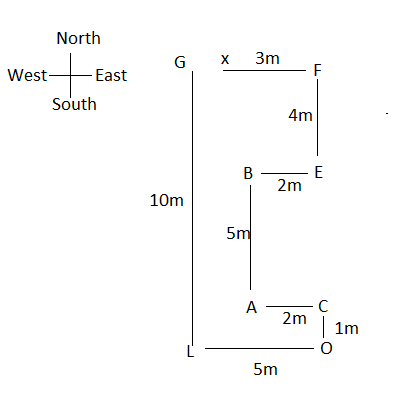Q-1) ANS- C) South east
Q-2) Ans – B) 2m
Q-3)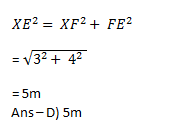Q:4-6)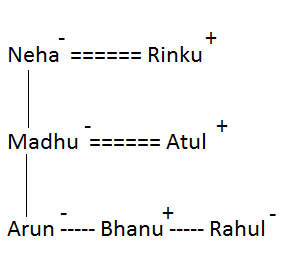Q-4) Ans – B) Three
Q-5) Ans – C) son in law
Q-6) Ans- D) Neha

Q: 7-9)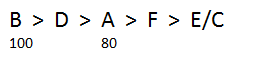Q-7) Ans- E) can not be determine
Q-8) Ans – E) 90
As the only 90kg  can be the weight because all other are more than 100 or less than 80
Q-9) Ans – c) F

Q-10) ANS - Only conclusion I follows
I)Some  Pens are Tab - follow
II)Only few Tab are Word – does not follow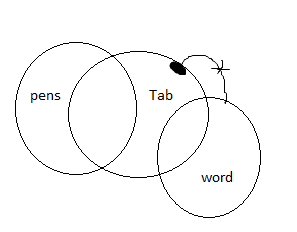### Disclaimer

अपनी वेबसाइट पर हम डाटा संग्रह टूल्स, जैसे की कुकीज के माध्यम से आपकी जानकारी एकत्र करते हैं ताकि आपको बेहतर अनुभव प्रदान कर सकें, वेबसाइट के ट्रैफिक का विश्लेषण कर सकें, कॉन्टेंट व्यक्तिगत तरीके से पेश कर सकें और हमारे पार्टनर्स, जैसे की Google, और सोशल मीडिया साइट्स, जैसे की Facebook, के साथ लक्षित विज्ञापन पेश करने के लिए उपयोग कर सकें। साथ ही, अगर आप साइन-अप करते हैं, तो हम आपका ईमेल पता, फोन नंबर और अन्य विवरण पूरी तरह सुरक्षित तरीके से स्टोर करते हैं। आप कुकीज नीति पृष्ठ से अपनी कुकीज हटा सकते है और रजिस्टर्ड यूजर अपने प्रोफाइल पेज से अपना व्यक्तिगत डाटा हटा या एक्सपोर्ट कर सकते हैं। हमारी Cookies Policy, Privacy Policy और Terms & Conditions के बारे में पढ़ें और अपनी सहमति देने के लिए Agree पर क्लिक करें।

Agree

Get free counselling session